Start typing, then use the up and down arrows to select an option from the list.# Half-Life Concept 3

Jules Bruno
116views
for reactions with second order, we use the following equation. So for second order rate law reactions, half life equals one over K times the initial concentration. So again, a sabo is initial concentration K equals our rate constant in since it's a second order process, the units for K would be polarity inverse times time, inverse T equals time. But if we're dealing with T have that equals half life now, what can we say about half life when it comes to the initial concentration and what happens to it as concentration decreases? We're looking at this formula for second order rate law processes. We say that the half life is dependent on initial concentration because it's found within the formula and it gets longer as the concentration decreases. So think about it. This number here is getting smaller and smaller. So one is getting divided by a smaller number, which means overall the number is larger. So you're half life is larger. So we'd expect that the half life for a second order process to increase over time, meaning that as time progresses it takes longer and longer for you to lose half of your substance. Okay, so it's a little bit weird. That's why when it comes to radioactive processes, we stick to first order processes um exclusively because they're more consistent. Right? So keep this in mind in terms of the formula. And how the formula relates to the initial concentration of reactant and how the concentration can affect the length of the half life for any second order rate law process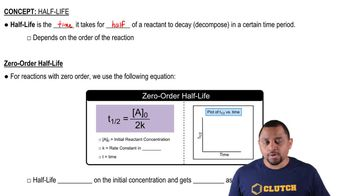02:17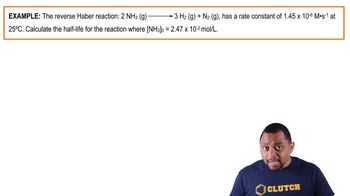01:19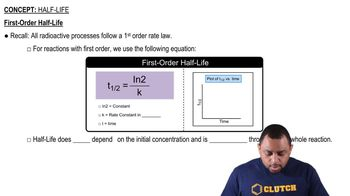01:4901:18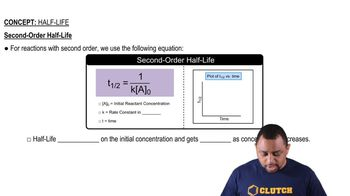01:44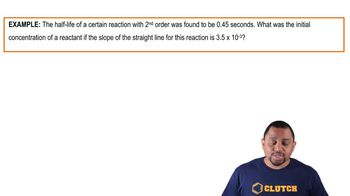01:52